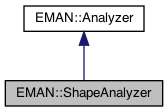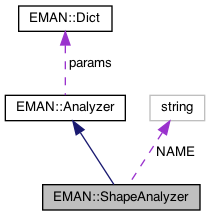EMAN2
EMAN::ShapeAnalyzer Class Reference

Shape characterization Computes a set of values characteristic of the shape of a volume. More...

`#include <analyzer.h>`

Inheritance diagram for EMAN::ShapeAnalyzer:[legend]
Collaboration diagram for EMAN::ShapeAnalyzer:[legend]

## Public Member Functions

ShapeAnalyzer ()

virtual int insert_image (EMData *image)
insert a image to the list of input images More...

virtual vector< EMData * > analyze ()
main function for Analyzer, analyze input images and create output images More...

string get_name () const
Get the Analyzer's name. More...

string get_desc () const
Get the Analyzer's description. More...

TypeDict get_param_types () const
Get Analyzer parameter information in a dictionary. More...Public Member Functions inherited from EMAN::Analyzer
Analyzer ()

virtual ~Analyzer ()

virtual int insert_images_list (vector< EMData * > image_list)
insert a list of images to the list of input images More...

virtual void set_params (const Dict &new_params)
Set the Analyzer parameters using a key/value dictionary. More...

virtual Dict get_params () const
Get the Reconstructor's parameters in a key/value dictionary. More...

## Static Public Member Functions

static AnalyzerNEW ()

## Static Public Attributes

static const string NAME = "shape"

## Protected Attributes

int verbose

vector< EMData * > retProtected Attributes inherited from EMAN::Analyzer
Dict params

vector< EMData * > images

## Detailed Description

Shape characterization Computes a set of values characteristic of the shape of a volume.

The first 3 values are distributions along X, Y and Z axes respectively, and are not orientation independent.

Date
12/29/2013
Parameters
 verbose Display progress if set, more detail with larger numbers

Definition at line 177 of file analyzer.h.

## ◆ ShapeAnalyzer()

 EMAN::ShapeAnalyzer::ShapeAnalyzer ( )
inline

Definition at line 180 of file analyzer.h.

180: verbose(0) {}

Referenced by NEW().

## ◆ analyze()

 vector< EMData * > ShapeAnalyzer::analyze ( )
virtual

main function for Analyzer, analyze input images and create output images

Returns
vector<EMData *> result os images analysis

Implements EMAN::Analyzer.

Definition at line 122 of file analyzer.cpp.

122 {
123 int verbose = params.set_default("verbose",0);
124 EMData *mx = new EMData(4,2,1); // result is 4 values
125 mx->to_zero();
126 ret.push_back(mx);
127
128 if (images.size()!=1) throw ImageDimensionException("Shape computation accepts only a single volume as input");
129 int nx=images->get_xsize();
130 int ny=images->get_ysize();
131 int nz=images->get_zsize();
132 if (nz==1 || ny==1 || nz==1) throw ImageDimensionException("Map must be 3-D");
133
134 if (verbose>0) printf("Shape size: %d %d %d\n",nx,ny,nz);
135
136 for (int z=0; z<nz; z++) {
137 for (int y=0; y<ny; y++) {
138 for (int x=0; x<nx; x++) {
139 int xx=x-nx/2;
140 int yy=y-ny/2;
141 int zz=z-nz/2;
142 float v=images->get_value_at(x,y,z);
143 mx->set_value_at(0,0,mx->get_value_at(0,0)+v*(xx*xx));
144 mx->set_value_at(1,0,mx->get_value_at(1,0)+v*(yy*yy));
145 mx->set_value_at(2,0,mx->get_value_at(2,0)+v*(zz*zz));
146 mx->set_value_at(3,0,mx->get_value_at(3,0)+v*(xx*xx+yy*yy+zz*zz));
147 // sum(m*r^2), in which r is the distance to the center. Used for minicircle classification
148 mx->set_value_at(0,1,mx->get_value_at(0,0)+v*abs(xx));
149 mx->set_value_at(1,1,mx->get_value_at(1,0)+v*abs(yy));
150 mx->set_value_at(2,1,mx->get_value_at(2,0)+v*abs(zz));
151 mx->set_value_at(3,1,mx->get_value_at(3,0)+v*(float)sqrt((float)(xx*xx+yy*yy+zz*zz)));
152 }
153 }
154 }
155 mx->mult(1.0f/(nx*ny*nz));
156
157 return ret;
158}
vector< EMData * > images
Definition: analyzer.h:117
type set_default(const string &key, type val)
Default setting behavior This can be achieved using a template - d.woolford Jan 2008 (before there wa...
Definition: emobject.h:569
EMData stores an image's data and defines core image processing routines.
Definition: emdata.h:82
vector< EMData * > ret
Definition: analyzer.h:216
EMData * sqrt() const
return square root of current image
#define ImageDimensionException(desc)
Definition: exception.h:166
#define y(i, j)
Definition: projector.cpp:1516
#define x(i)
Definition: projector.cpp:1517

## ◆ get_desc()

 string EMAN::ShapeAnalyzer::get_desc ( ) const
inlinevirtual

Get the Analyzer's description.

Returns
The Analyzer's description.

Implements EMAN::Analyzer.

Definition at line 195 of file analyzer.h.

196 {
197 return "Experimental. Computes a set of values characterizing a 3-D volume. Returns a 3x2x1 image containing X, Y and Z axial distributions using axis squared and axis linear weighting.";
198 }

## ◆ get_name()

 string EMAN::ShapeAnalyzer::get_name ( ) const
inlinevirtual

Get the Analyzer's name.

Each Analyzer is identified by a unique name.

Returns
The Analyzer's name.

Implements EMAN::Analyzer.

Definition at line 190 of file analyzer.h.

191 {
192 return NAME;
193 }
static const string NAME
Definition: analyzer.h:212

References NAME.

## ◆ get_param_types()

 TypeDict EMAN::ShapeAnalyzer::get_param_types ( ) const
inlinevirtual

Get Analyzer parameter information in a dictionary.

Each parameter has one record in the dictionary. Each record contains its name, data-type, and description.

Returns
A dictionary containing the parameter info.

Implements EMAN::Analyzer.

Definition at line 205 of file analyzer.h.

206 {
207 TypeDict d;
208 d.put("verbose", EMObject::INT, "Display progress if set, more detail with larger numbers");
209 return d;
210 }

References EMAN::EMObject::INT, and EMAN::TypeDict::put().

## ◆ insert_image()

 virtual int EMAN::ShapeAnalyzer::insert_image ( EMData * image )
inlinevirtual

insert a image to the list of input images

Parameters
 image
Returns
int 0 for success, <0 for fail

Implements EMAN::Analyzer.

Definition at line 182 of file analyzer.h.

182 {
183 images.push_back(image);
184 if (images.size()>1) { printf("ShapeAnalyzer takes only a single image\n"); return 1; }
185 return 0;
186 }

References EMAN::Analyzer::images.

## ◆ NEW()

 static Analyzer * EMAN::ShapeAnalyzer::NEW ( )
inlinestatic

Definition at line 200 of file analyzer.h.

201 {
202 return new ShapeAnalyzer();
203 }

References ShapeAnalyzer().

## ◆ NAME

 const string EMAN::ShapeAnalyzer::NAME = "shape"
static

Definition at line 212 of file analyzer.h.

Referenced by get_name().

## ◆ ret

 vector EMAN::ShapeAnalyzer::ret
protected

Definition at line 216 of file analyzer.h.

Referenced by analyze().

## ◆ verbose

 int EMAN::ShapeAnalyzer::verbose
protected

Definition at line 215 of file analyzer.h.

Referenced by analyze().

The documentation for this class was generated from the following files: